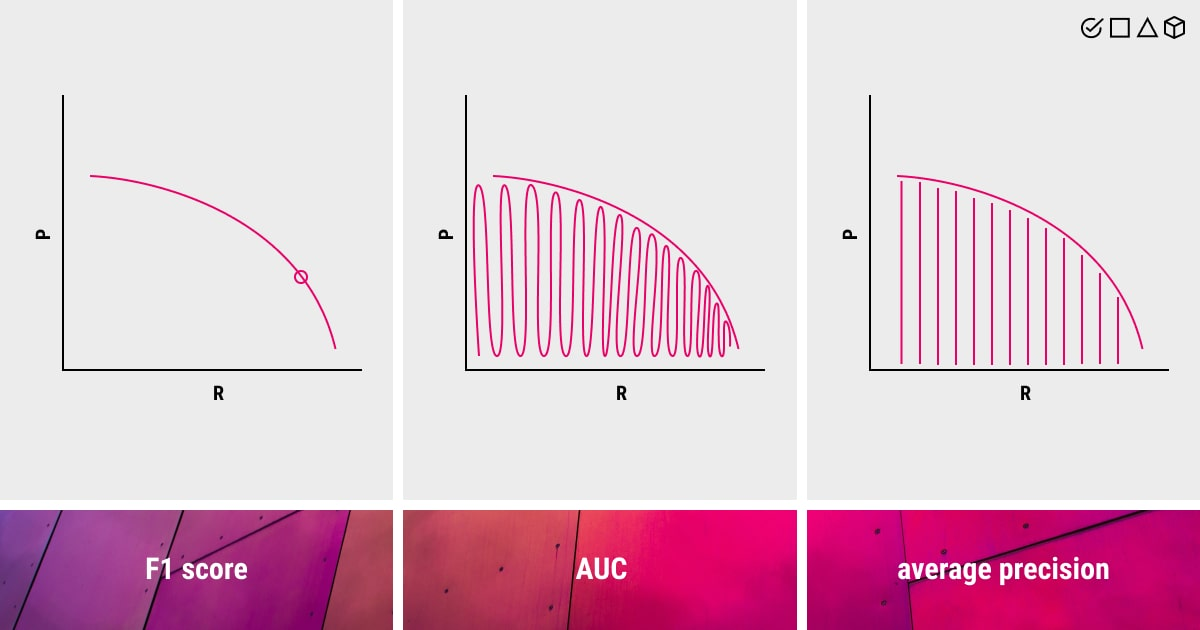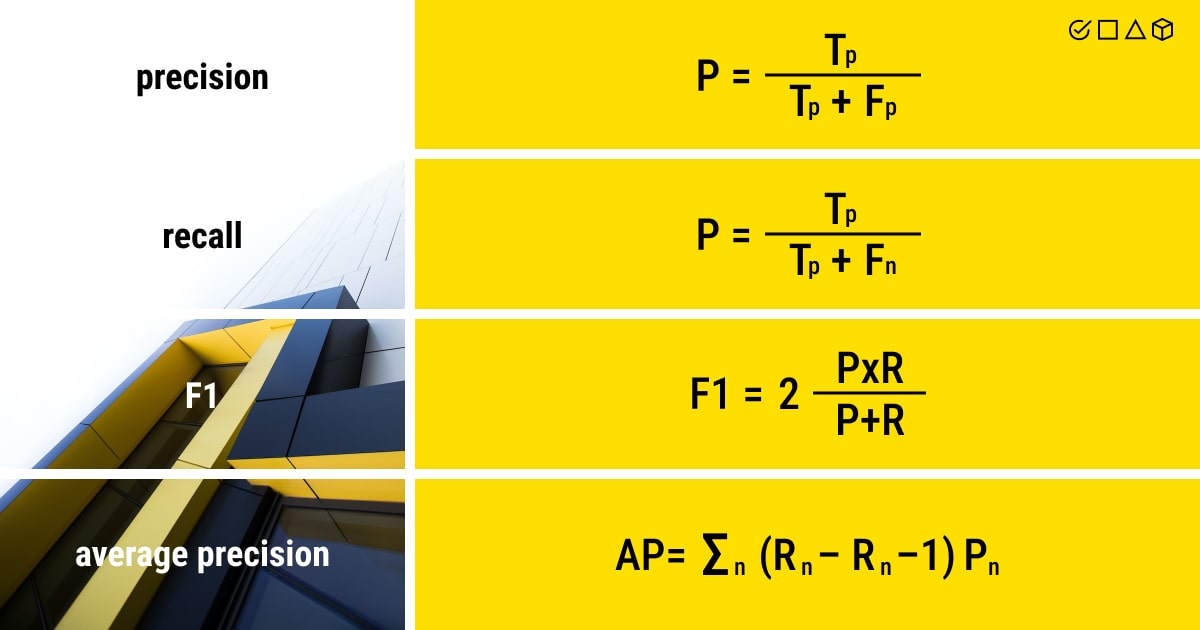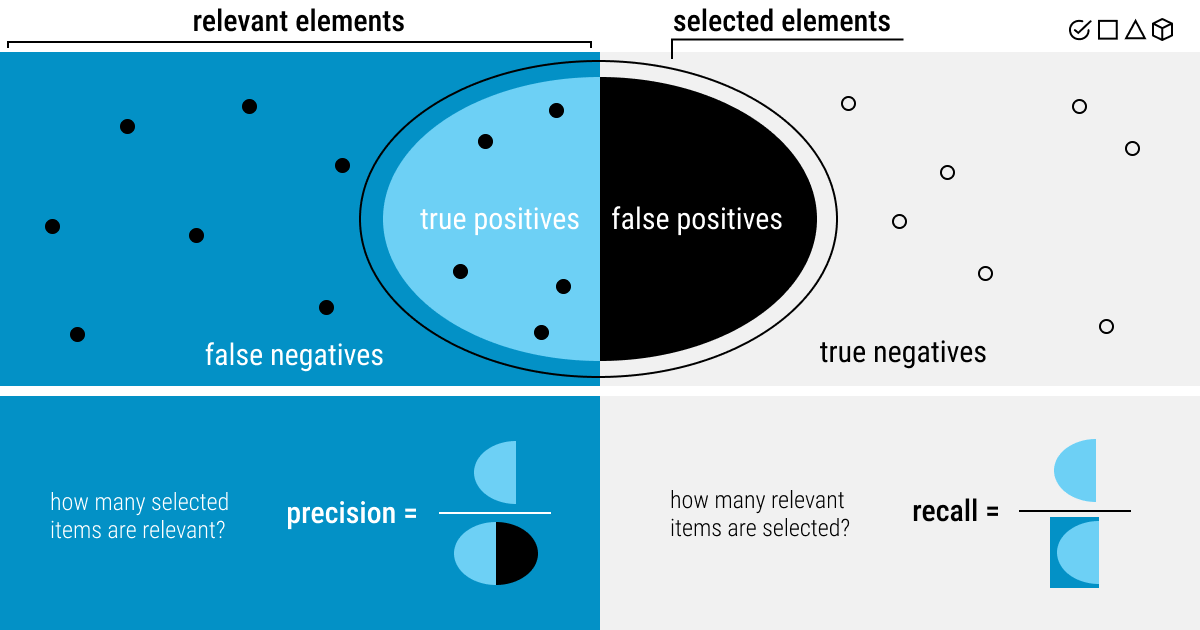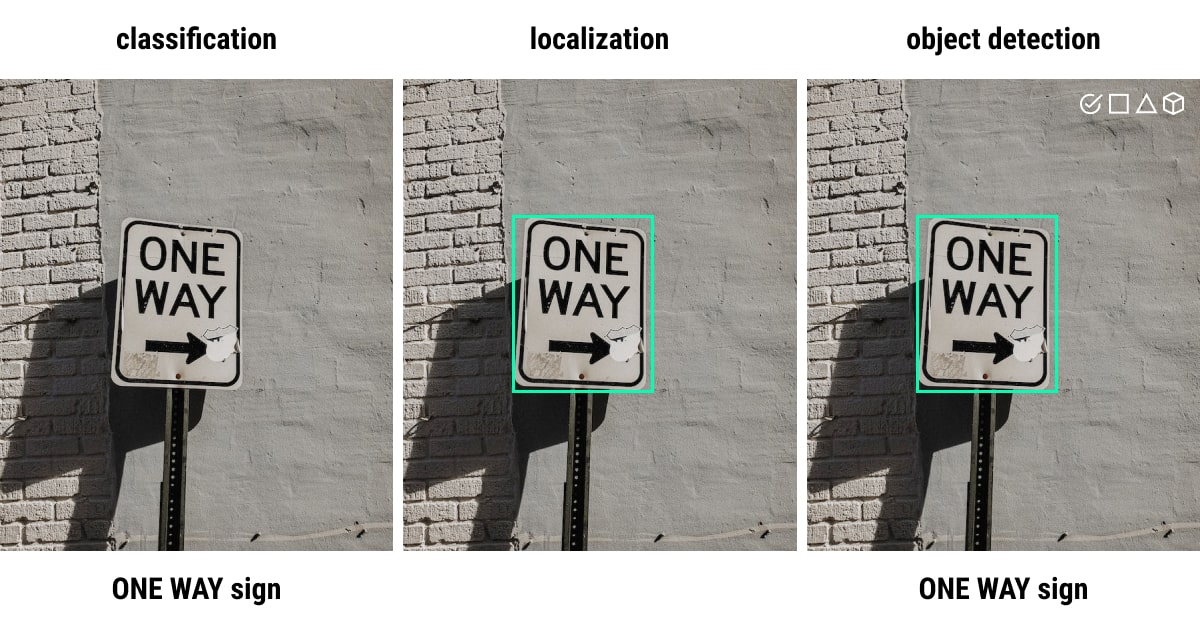Most people mistakenly assume that mean average precision, or simply mAP, is directly related to the average of precision. Although it seems quite logical, in practice it is not the case. The underlying principle of mAP is far more complex.

Mean average precision is a well-known evaluation metric for object detection in computer vision (CV) (i.e., localization and classification tasks). Localization pinpoints an object’s position using, for example, bounding box coordinates, and classification identifies it (whether it’s a dog or a cat). Therefore, the performance of object detection algorithms, segmentation systems, and information retrieval tasks, is frequently examined using the mAP metric.

Many object detection models employ mean average precision to assess their performance before releasing the final results, including Faster R-CNN, MobileNet SSD, and YOLO. A number of benchmark tasks, including Pascal, VOC, COCO, and others, also make use of the mAP. More specifically, recall values between 0 and 1 are used to determine the average precision (AP) values.

Let’s take a look now at the mAP formula to give you a better idea of this metric in computer vision:

• Q is the total number of queries in the set
• AveP(q) is the average precision (AP) for a given query Q

For a particular query (Q), we compute its associated average precision (AP), and the mean of all these AP scores would give us a single value, termed the mean average precision (mAP), which quantifies how well our model performs the query. This is basically what the mAP formula is all about.

The following sub-metrics form the backbone of the formula for the ultimate mAP accuracy:

• Confusion Matrix
• Intersection over Union (IoU)
• Recall
• Precision

However, to fully understand mean average precision, one must first grasp the idea of “precision” and “recall”. This understanding calls for knowledge of true/false positives and true/false negatives in the context of object detection, which we’ll discuss shortly.

When talking about object detection, what we mean by that is essentially the task of identifying target objects in the images or videos and classifying them into relevant classes, using machine learning or deep learning techniques. A good example of this would be tumor detection in medical images: you need annotated medical image data to train an object detection model (of your choice) to recognize tumors and classify them automatically and accurately.

But the question is: how do we decide which model works best for a given task? Having the opportunity to directly measure how each model performs across images in our test set, across classes, and at various confidence criteria could truly save the day. So we say: bring in the mAP! But we must first spend some time studying the precision-recall curve to comprehend the mean average precision.

## The Precision-Recall Curve in Machine Learning mAPAn example of the precision-recall curve

When the classes are severely unbalanced, precision-recall is a helpful indicator of prediction success. Precision in information retrieval refers to how relevant the results are, whereas recall measures the number of outcomes that are actually relevant.

Examining the trade-off between precision and recall values at various thresholds is important due to the role of both of these metrics. This curve helps choose the ideal threshold to optimize both metrics. High recall and high precision are both represented by a high area under the curve, where high accuracy is correlated with a low false positive rate and high recall is correlated with a low false negative rate. High scores for both indicate that the classifier is producing results with high accuracy and a high percentage of good findings (high recall).

The precision-recall curve requires the following inputs to be created:

• The ground-truth labels.
• The prediction scores of the samples.
• A few thresholds to turn the prediction scores into class labels.

The majority of the suggested labels from a system with high recall but low accuracy are inaccurate when compared to the training labels. The reverse is true for a system that has high recall but low accuracy: it produces very few results, yet most of the labels match the training labels. The ideal system will provide numerous results, all of which will be accurately categorized, and have a high recall and accuracy.

How do you get the precision-recall curve? You may get it by graphing the accuracy and recall values of the model as a function of the model’s confidence score threshold. In this case, precision is associated with correct prediction and shows to what extent the model’s positive predictions may be trusted. Recall, in turn, measures the model’s capacity to identify positive samples and demonstrates any predictions that a model shouldn’t have missed. Important note: precision and recall cannot be used independently of one another, which is why we use a curve.

The reason for this is simple. When there’s a high recall but low accuracy, your model produces many false positives (negative samples are categorized as positive), even though it classifies the positive samples correctly. Conversely, with high accuracy but low recall, your model is only accurate when classifying samples as positive; but it might do so with only some of the positive samples.

To sum up, the precision-recall curve captures the trade-off between the two criteria and maximizes their combined impact. As a result, we get a clearer picture of the model’s overall accuracy in performing object detection. Such a curve is commonly used in binary classification to analyze the output of a given classifier.

### The Key Metrics of Mean Average Precision

As we’ve mentioned above, there are certain metrics and sub-metrics of mean average precision that form the basis of this evaluation metric used in computer vision. They include confusion matrix, intersection over union (IoU), recall, and precision. Let’s discuss each of them in more detail!

First, you need to create a confusion matrix. Here are some important components that will help you accomplish this task (hint: we’ve mentioned them in the precision-recall curve):

• True positives (Tp): The model properly predicted a label and matched the ground truth (i.e., the accuracy of the training set’s classification). The model has detected the right type of object and its correct location.
• True negatives (Tn): The model is not a part of the ground truth, and neither does it forecast the label. The model has correctly predicted the wrong class.
• False positives (Fp): A label was predicted by the model, however, it is not a part of the ground truth. The model has detected an object that is not there or provided a wrong label.
• False negatives (Fn): A label is not predicted by the model, but it is a component of the ground truth. The object hasn’t been detected by the model.

Second, the concept of intersection over union (IoU) describes the relationship between the expected bounding box coordinates and the actual box. Higher IoU implies a closer match between the anticipated and actual bounding box coordinates. Basically, you measure the correctness with IoU.

Want to learn more about the bounding boxes and other data annotation types and tools? We have an entire article prepared for you about the basics of data labeling. Alternatively, you can reach out to our team at Label Your Data and let us do the task for you!

After our model has produced its predictions, we examine each one and give it one of the aforementioned labels. This allows us to measure precision and recall. The formulas for both (and more) are as follows:

• Precision is viewed as the number of true positives (Tp) over the number of Tp plus the number of false positives (Fp);
• Recall is seen as the number of Tp over the number of Tp plus the number of false negatives (Fn).
• F1 score is the harmonic mean of both precision and recall.
• Average precision(AP) calculates the weighted average of precision at each threshold, with the increase in recall from the preceding threshold serving as the weight.

*All the formulas are illustrated below.

Despite first appearing random, these formulas make a lot of sense. In a nutshell, recall and precision provide crucial insights into the object detection model performance. With precision, we can better understand how many correct predictions are out of all the results. Recall reveals how many examples of a given class in the dataset has the model discovered. This is exactly how you can get a complete view of the model’s performance.

In reality, both precision and recall are applied in a wide range of cases and are not restricted to object detection or even computer vision.Illustrating the formulas: Precision, Recall, F1, and Average Precision

## Calculating mAP to Assess the Model’s Performance

Now it’s time to summarize all the gained knowledge and proceed with the actual calculations of the mAP to evaluate the model’s performance in object detection or other tasks.

The weighted mean of precision at each threshold is used to determine the average precision score (AP), while the weight itself represents the increase in recall from the previous threshold. Therefore, the average of AP of each class is known as the mean average precision (mAP). However, the meaning of AP and mAP vary depending on the context. For example, AP and mAP are equivalent in the evaluation report for the COCO object detection task.

We have compiled all the necessary steps you need to take to measure mAP into one list, so you can better process and structure all the information we provided in this article:

• Using the model, provide the prediction scores.
• Create class labels by converting the prediction scores.
• Calculate the confusion matrix, including Tp, Tn, Fp, and Fn.
• Measure the precision and recall metrics for different IoU thresholds.
• Plot them against each other.
• Do the calculations of the area under the precision-recall curve.
• Measure the average precision score (AP).
• Calculate the mAP by using AP for each class and the average over a number of classes.The key elements of confusion matrix

### Applications of Mean Average Precision for Object Detection

Object detection seems an easy CV task at first sight: you have a labeled training dataset and a model to train it with. However, once your model is trained, you spend hours evaluating its performance to make sure you get accurate and credible results. Evaluations and tons of metrics might leave you frustrated in the end because the expectations were not met.

In this particular case, you need to master only one metric to benchmark model performance, that is mean average precision (mAP). As a common metric for assessing an object detection model’s precision, mAP has gained traction in machine learning and deep learning. Yet, it’s still difficult to disentangle errors in object detection and instance segmentation from mAP.

#### COCO Evaluator & mAP

Recent research publications typically solely present findings for the COCO dataset. The computation in COCO mAP uses a 101-point interpolated AP definition. AP for COCO is the average over many IoU (the minimum IoU to consider a positive match). One can assess a COCO evaluator can be used, for instance, to assess a YOLO object detection model.

## Let’s Recap! How Crucial Is Mean Average Precision in Machine Learning?mAP is a crucial metric for achieving accurate object detection

Don’t be so easily misled by the term “mean average precision” because you might fall into the trap!

In this article, we tried to explain the tricky concept of mean average precision, a common practice for model evaluation in computer vision, particularly for object detection purposes. However, we understand how confusing the abundance of mathematics and formulas might be for you. Let’s summarize what we’ve covered about mAP so far:

• In computer vision, mean average precision (mAP) is used as a standard metric to evaluate the accuracy of object detection algorithms.
• In the precision-recall curve, precision is responsible for the accuracy of predictions, while recall measures how many predictions match the ground truth.
• The intersection over union (IoU), which assigns a score to the identified objects in an object detection model, serves as the threshold.
• The mAP is calculated once the AP for each class in the dataset has been determined.

As such, mAP evaluation is an essential step in machine learning to build accurate object detection models and ensure it provides the most reliable results. It offers a valuable assessment of how well the model performs. However, you should never forget that the accuracy of an ML model always starts with annotated data first.

If you need to make your data meaningful to train your model, contact our team to get professional help with data annotation. Expand your opportunities in AI with Label Your Data!by Yuliia Kniazieva
on September 1, 2022.Get Notified ⤵

Receive weekly email each time we publish something new: